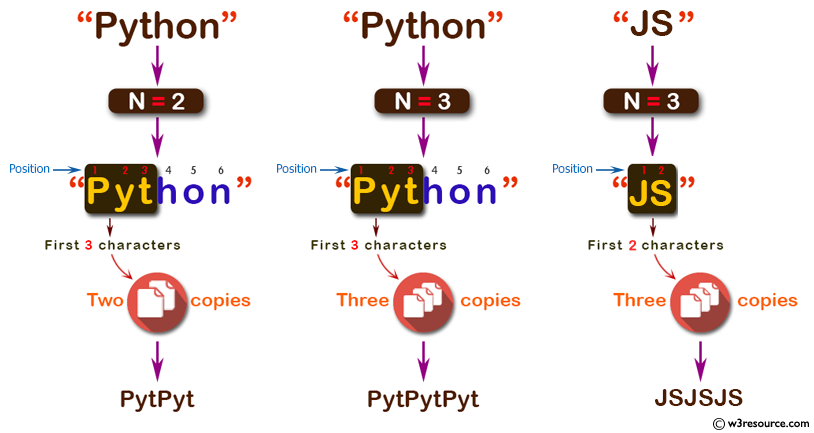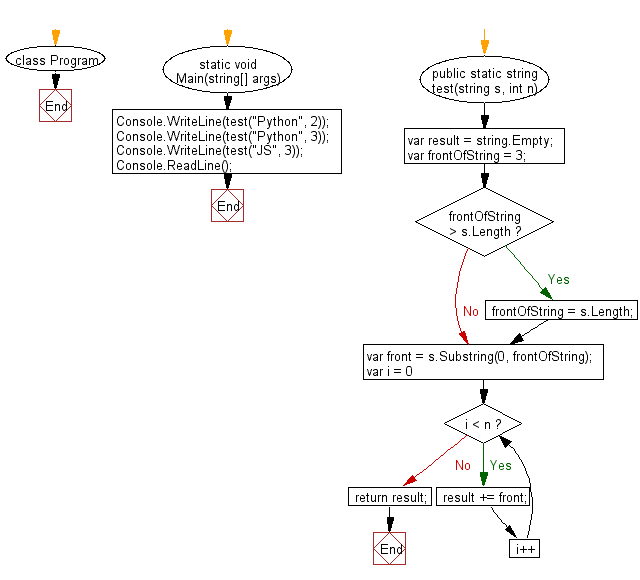﻿ C# - Make n copies of the first 3 characters of a string# C# Sharp Basic Algorithm Exercises: Create a new string which contain the n copies of the first 3 characters of a given string

## C# Sharp Basic Algorithm: Exercise-26 with Solution

Write a C# Sharp program to create a new string which contain the n (non-negative integer ) copies of the first 3 characters of a given string. If the length of the given string is less than 3 then return n copies of the string.

Pictorial Presentation:Sample Solution:-

C# Sharp Code:

``````using System;
namespace exercises
{
class Program
{
static void Main(string[] args)
{
Console.WriteLine(test("Python", 2));
Console.WriteLine(test("Python", 3));
Console.WriteLine(test("JS", 3));
}

public static string test(string s, int n)
{
var result = string.Empty;
var frontOfString = 3;

if (frontOfString > s.Length)
frontOfString = s.Length;

var front = s.Substring(0, frontOfString);

for (var i = 0; i < n; i++)
{
result += front;
}
return result;
}
}
}
```
```

Sample Output:

```PytPyt
PytPytPyt
JSJSJS```

Flowchart:C# Sharp Code Editor:

Improve this sample solution and post your code through Disqus

What is the difficulty level of this exercise?

Test your Programming skills with w3resource's quiz.

﻿